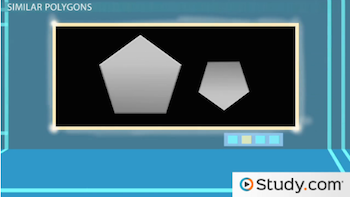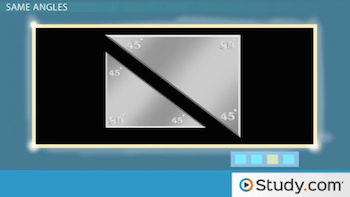# Similar Polygons: Definition and Examples

An error occurred trying to load this video.

Try refreshing the page, or contact customer support.

Coming up next: The Transitive Property of Similar Triangles

### You're on a roll. Keep up the good work!

Replay
Your next lesson will play in 10 seconds
• 0:08 Polygons
• 0:50 Similar Polygons
• 1:39 Same Angles
• 4:31 Proportional
• 6:58 Lesson Summary

Want to watch this again later?

Timeline
Autoplay
Autoplay
Speed

#### Recommended Lessons and Courses for You

Lesson Transcript
Instructor: Yuanxin (Amy) Yang Alcocer

Amy has a master's degree in secondary education and has taught math at a public charter high school.

Watch this video to learn what makes two polygons similar to each other. You'll also learn the one distinguishing aspect of similar polygons and see an example of how to compare two polygons.

## Polygons

To begin, let's talk about polygons and what they are. Polygons are defined as two-dimensional shapes with straight sides. If you take a pen or pencil starting at one point on your piece of paper and, without lifting your pen or pencil, you draw straight lines until you return to your starting point, you will have drawn a polygon. Our most common shapes, such as triangles, squares, and rectangles, are all polygons. Circles are not, because they have curved sides. A star is a polygon because all its sides are straight.

## Similar PolygonsWhen we say that two polygons are similar, we mean that the only difference between them is size. If one is the exact same shape as the other, just smaller or larger, then they are similar.

Sometimes, one of the shapes may be flipped around or rotated, but the shapes are still similar. It's like you cutting two similar triangles out of a piece of paper. You rotate and flip one of them. Now the shapes look different at first glance, but you know that they are still similar polygons. When working with problems, keep this point in mind. Look at the two shapes and imagine flipping and rotating them to see if they really are different or if they are similar.

## Same Angles

Now, imagine taking a shape and stretching it out to make it bigger. What do you notice about the shape? You notice that it keeps it proportions. You also see that you can place your original shape inside the larger shape and match each of the angles together. You see that the only difference is the length of the sides.

What you have just found is that the one distinguishing aspect of similar polygons is that all the angles remain the same. No matter how much you increase or decrease the size of the shapes, the angles will always remain the same measurement. If one angle in a triangle measures 60 degrees, then the same angle in a similar triangle will also measure 60 degrees.

Let's try comparing two triangles together to see if they are similar. Look at these triangles. When you first look at these two triangles, what do you see? Can you tell that they are similar at first glance? What I do is I imagine rotating one of them in my head to see what happens, to see if that shape starts to resemble the other shape. I pick the smaller one to rotate. I see that if I rotate the shape 180 degrees, or halfway around a circle, that the smaller one looks a lot like the bigger one. I remember that the one distinguishing mark for similar polygons is that all the matching angles must equal each other, so I look at my angles to see if they are the same. I see that my two little angles are the same and my larger angle is also the same. What do you know? These are similar triangles!Some problems you encounter might ask you to find the angle of one shape given that the shape is similar to another shape that has the angle marked. In this case, you would rotate or flip the shape until they look similar, then you can match the angles to each other to find the angle measurement. For example, say you were given two triangles like these.

You are told that the triangles are similar and the problem is asking you to find the measure of angle A. What do you do? First, you look to see if you have to rotate or flip. You see that the two triangles already look alike, so now all you have to do is match the angles. Angle A is the top angle in the smaller triangle, so what is the top angle in the larger triangle? You see that it is 75, so the answer is 75 degrees.

To unlock this lesson you must be a Study.com Member.

### Register to view this lesson

Are you a student or a teacher?

### Unlock Your Education

#### See for yourself why 30 million people use Study.com

##### Become a Study.com member and start learning now.
Back
What teachers are saying about Study.com

### Earning College Credit

Did you know… We have over 160 college courses that prepare you to earn credit by exam that is accepted by over 1,500 colleges and universities. You can test out of the first two years of college and save thousands off your degree. Anyone can earn credit-by-exam regardless of age or education level.